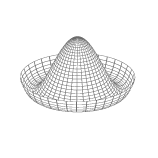4:11 AM
@EmilioPisanty wait 'til you get to 666,666... That will be evil.4:24 AM
0I've seen two hot network questions in the past two days where one of the highly upvoted answers was a variation on "you did your measurement wrong". In both cases, this answer was incorrect--the measurement was right, the simplest physical model was wrong, and there was better physical model th...

2 hours later…6:12 AM
Hi All..

6 hours later…11:53 AM
does $\Lambda^T\Lambda=I$ lead to $\Lambda\Lambda^T=I$? If so, how to prove it?
I think this should be the case if $\Lambda$ is an element of 0(3) because a group element should satisfy $\Lambda^{-1}\Lambda=I$ and $\Lambda\Lambda^{-1}=I$, but I don't know how to prove it.

1 hour later…2 hours later…3:34 PM
Good morning
Consider a semi-Riemannian manifold $\zeta^{2,2}$ with metric, $g=\frac{dxdy}{xy}+\frac{dudv}{v-uv}.$ How could you define a 3-dimensional "slice" of $\zeta^{2,2}$? I realize the question is ill-posed as it stands, because I have to precisely define what I mean by "slice", but I'm struggling to precisely define itin a Lorentzian setting you'd be looking for a foliation by Cauchy surfaces, but I have no idea why you're looking at signature (2,2) or what sort of "slice" you're loking forokay. Also do you know how to express $\Bbb R^{3,1}$ as a product manifold?3:51 PM
I feel we've had this conversation before :P
$\mathbb{R}^{n,m} = \mathbb{R}^{p,q} \times \mathbb{R}^{s,t}$ where $p+s = n$ and $q +t = m$.oh right, sorry. $\Bbb R^{1,0} \times \Bbb R^{1,0} \times \Bbb R^{1,1}$
is that correct^?sure, that's one way to do it4:38 PM
Hey All..

2 hours later…6:50 PM
I'm confused that this article says wool is a conductive material: scientificamerican.com/article/…
If it's conductive, why doesn't it behave like a metal and doesn't get back the electrons quickly?7:05 PM
1So, I'm reading through Polyakov's paper from 1974, "Particle Spectrum in Quantum Field Theory." I'm trying to work through all of the steps and properly understand everything. For context, this is one of the papers along with 't Hooft's that the name 't Hooft-Polyakov monopoles came from. The pa...

Progress7:58 PM
Hello everyone
I've been stumped trying to prove the rolling without slipping condition for a particular special case. Anyone interested in helping me out?@aderchox Wool is a pretty good insulator, but not as good as rubber. The article should probably say “electronegative,” but it’s for kids.8:25 PM
@rob Yeah guessed that might be the reason, but couldn't be sure about it. Thanks a lot.